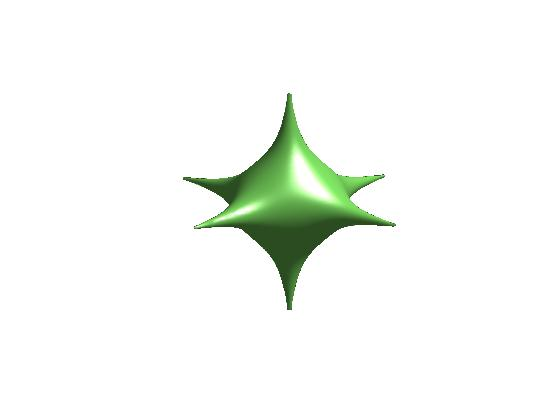## A little love for MATLAB – plotting algebraic surfaces

December 15th, 2008 | Categories: matlab | Tags:

Back in February I blogged about a little Mathematica demonstration I wrote which plotted several heart shaped algebraic surfaces. One or two people wrote in the comments section asking how to do such plots in MATLAB and I thought that it was about time I came up with the code.
 %code to plot a heart shape in MATLAB %set up mesh n=100; x=linspace(-3,3,n); y=linspace(-3,3,n); z=linspace(-3,3,n); [X,Y,Z]=ndgrid(x,y,z); %Compute function at every point in mesh F=320 * ((-X.^2 .* Z.^3 -9.*Y.^2.*Z.^3/80) + (X.^2 + 9.* Y.^2/4 + Z.^2-1).^3); %generate plot isosurface(F,0) view([-67.5 2]); 

It’s not really the right time of year for hearts though is it? So let’s plot a star instead.%Code to plot a 3D star in MATLAB %set up mesh n=200; x=linspace(-2,2,n); y=linspace(-2,2,n); z=linspace(-2,2,n); [X,Y,Z]=ndgrid(x,y,z); %Compute function at every point in mesh F=X.^2+Y.^2+Z.^2+1000*(X.^2+Y.^2).*(X.^2+Z.^2).*(Y.^2+Z.^2); %generate plot isosurface(F,1); axis off; view([-48 18]); 

For more examples of algebraic surfaces see the Algebraic Surfaces Gallery.

1. Happy Valentine’s Day!

This was a far as I got making the heart pink:

%code to plot a heart shape in MATLAB
%set up mesh
n=100;
x=linspace(-3,3,n);
y=linspace(-3,3,n);
z=linspace(-3,3,n);
[X,Y,Z]=ndgrid(x,y,z);
%Compute function at every point in mesh
F=320 * ((-X.^2 .* Z.^3 -9.*Y.^2.*Z.^3/80) + (X.^2 + 9.* Y.^2/4 + Z.^2-1).^3);
%generate plot
p = patch(isosurface(F,0));
set(p,’FaceColor’, ‘red’,’EdgeColor’, [1 .5 .5]);
view([-67.5 2]);